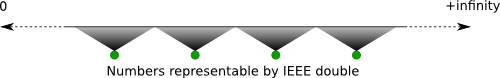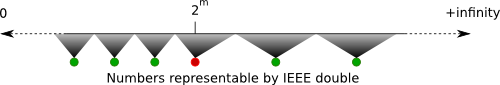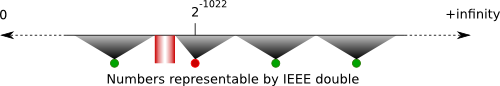# 2011/02/03

## Bitten by floating point numbers again

When I read about PHP hanging while parsing 2.2250738585072011e-308, I did what most language implementers must have done---typed in the number to the Gauche prompt. Gauche wasn't vulnerable to this number. Good.

It is reported that PHP problem was that Intel's 80bit extended floating point calculation was used where IEEE double precision calculation should have been used. Like PHP, Gauche refers to Clinger's paper (PS), but ours is rather naive implementation of Clinger's `AlgorithmR` and using exact integer arithmetic, thus we have nothing to do with extended precision calculation.

So when I heard about Java hangs reading 2.2250738585072012e-308 shortly after, I didn't bother checking. Ah the same problem again.

Well, it wasn't.

Jens Thiele reported that Gauche had the same issue as Java.

```gosh> 2.2250738585072012e-308
;; => hangs
```

Let's see what is happening. For simplicity we only consider positive numbers, but the discussion applies to negative numbers as well by reverting comparisons (e.g. less than -> greater than).

The floating point number reader must map the input real number to the closest IEEE double precision number ("IEEE double" from now on). In most cases, each IEEE double covers the region of real numbers between 1/2 LSB greater than and 1/2 LSB less than the exact real number it represents, as shown below.If the input falls on the exact center of two IEEE doubles, we use round-to-even rule.

There are exceptions on the number 2^m. The adjacent IEEE double below 2^m is closer than the one above, since we switch exponent below this boundary, so the numbers below it have greater precision. Those IEEE doubles on the boundary covers between 1/2 LSB greater than, but only 1/4 LSB less than, the exact number it represents. Clinger's paper, and thus Gauche, correctly account this boundary case.However, there is one exception among these exceptions: The minimum normalized number, 2^(-1022). In this case, the adjacent IEEE double below is just as far apart as the one above, since we don't have more precision anymore.

Our implementation of `AlgorithmR` missed this condition, which created a gap in the coverage.The number 2.2250738585072012e-308 falls into this gap. When the algorithm refines approximation, it thinks the input number is too small to round to 2^(-1022), so it decreases the estimate. Then it finds the input is too large to round down, so it increases the estimate. Repeat ad infinitum.

This anormality only occurs here, since no denormalized numbers satisfy our boundary condition check for the 2^m cases.

The fix is just one line.

```--- src/number.c        (revision 7350)
+++ src/number.c        (working copy)
@@ -3543,6 +3543,7 @@
case -1: /* d2 < y */
if (Scm_NumCmp(m, SCM_2_52) == 0
&& sign_d < 0
+                && k > -1074
&& Scm_NumCmp(Scm_Ash(d2, 1), y) > 0) {
goto prevfloat;
} else {
```

Tag: Flonums